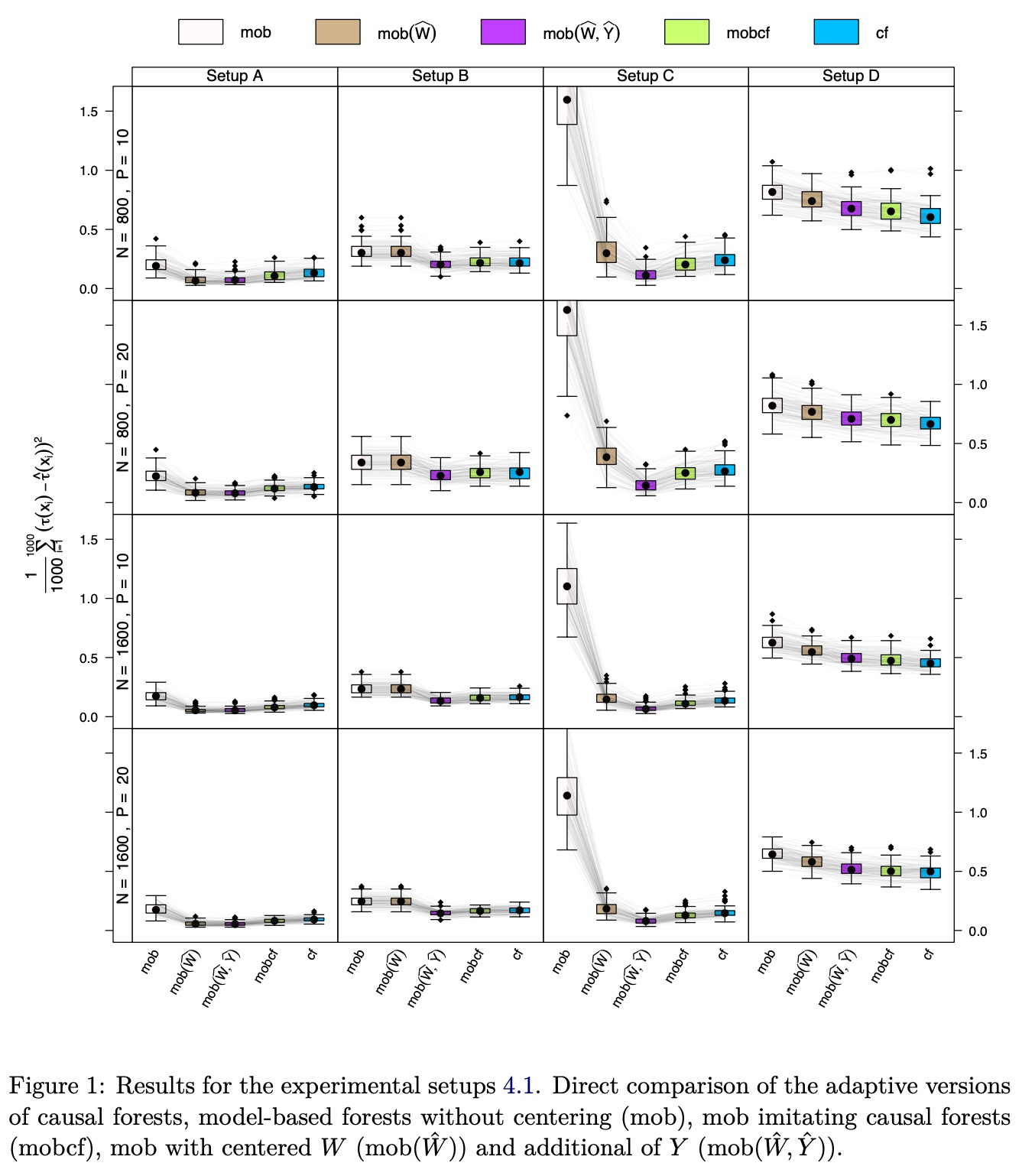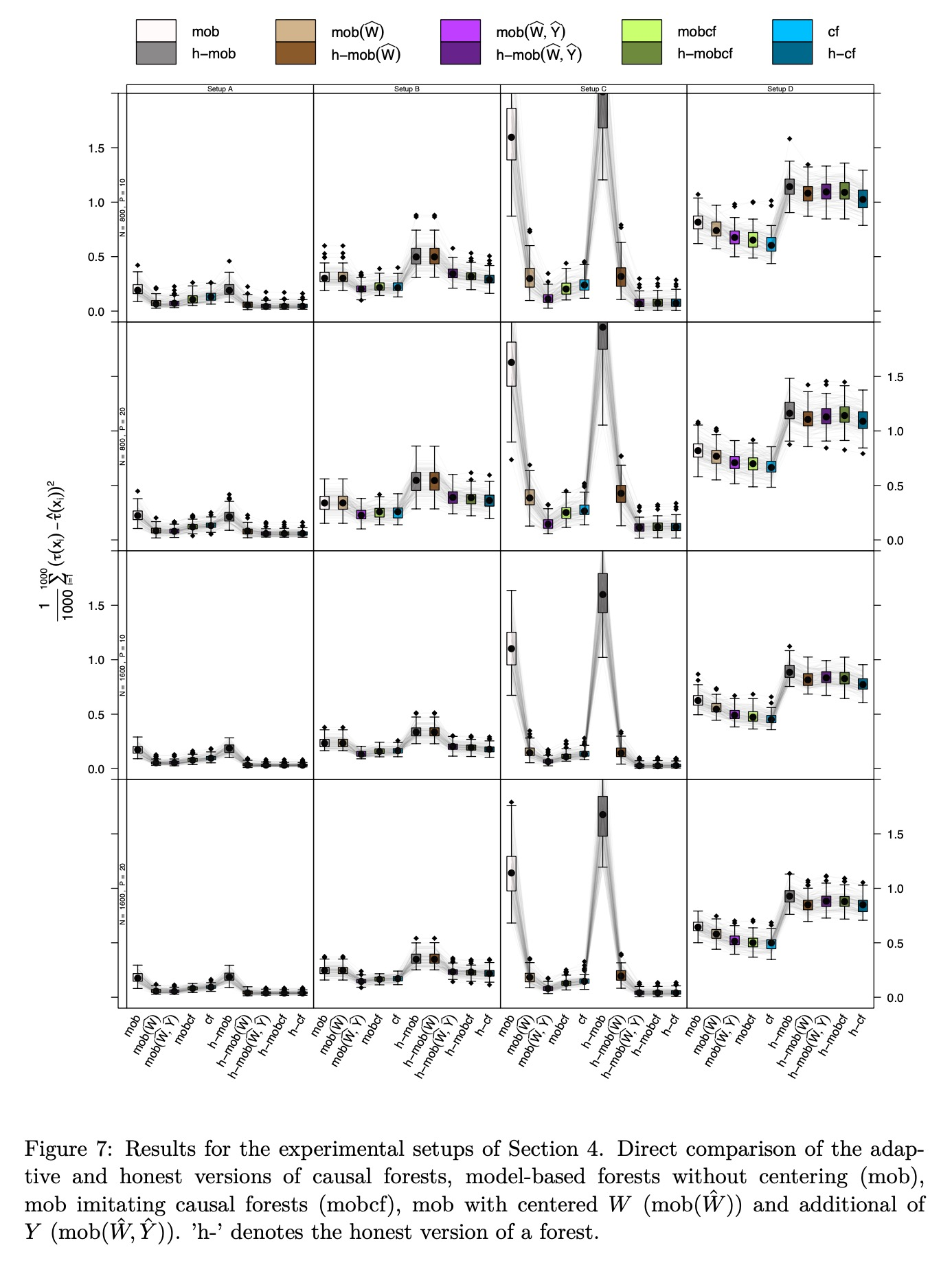TL;DR,

# 问题定义 & 模型

$$Y = \mu({\rm{X}}) + \tau({\rm{X}}) W +\phi Z$$

$${\Bbb{E}(Y|{\rm{X}}=x)}=\mu(x) +\tau(x)\pi(x) =:m(x)$$

$$0 < \pi(x)={\sf{P}}(W=1|{\rm{X}}={\rm{x}})={\Bbb{E}}(W|{\rm{X}}={\rm{x}}) < 1$$

$$\ell_\text{cf}(\tau) = \frac{1}{2}\Big(Y-\hat{m}({\rm{x}})-\tau({\rm{x}})\big(w-\hat{\pi}({\rm{x}})\big)\Big)^2$$

$$s_\text{cf}(\tau)=\frac{\partial \ell_\text{cf}(\tau)}{\partial \tau} = \Big( Y - \hat{m}({\rm{x}}) - \tau\big(w-\hat{\pi}({\rm{x}})\big) \Big) \big(w - \hat{\pi}({\rm{x}})\big) = 0$$

$$s_\text{cf}(\hat{\tau})=\Big( Y - \hat{m}({\rm{x}}) - \hat{\tau}\big(w-\hat{\pi}({\rm{x}})\big) \Big) \big(w - \hat{\pi}({\rm{x}})\big)$$

$$\ell_\text{mob}(\mu,\tau) = \frac{1}{2}\Big(Y - \mu({\rm{x}}) - \tau({\rm{x}})w\Big)^2$$

$$s_\text{mob}(\hat{\mu},\hat{\tau}) = (Y - \hat{\mu} - \hat{\tau} w)(1, w)^\mathsf{T}$$
（因为这篇文章中，我不太关心model-based forest的具体算法，所以就不做具体展开了）

$$\hat{\tau}({\rm{x}}) = \underset{\tau}{\arg\min} \sum_i^n \alpha_i^\text{cf}({\rm{x}})\ell_{\text{cf},i}(\tau)\ \big(\hat{\mu}({\rm{x}}), \hat{\tau}({\rm{x}})\big)^{\mathsf{T}} = \underset{\mu, \tau}{\arg\min} \sum_i^n \alpha_i^\text{mob}({\rm{x}})\ell_{\text{mob},i}(\mu,\tau)\$$

# 实验 & 结论• Setup A，比较复杂的confounding，model-based forest很难处理这种情况（该算法常用于随机数据），对$W$做local centering是有效的，同时对$W$和$Y$做并没有进一步提升效果，causal forest有相近的表现，但是会稍微差一点

• Setup B，完全随机，同时对$W$和$Y$做local centering会效果更好，即使此时并没有调整confounding的必要

• Setup C，强confounding，没有local centering的模型效果非常差，对于$W$做会有很大改进，同时对$W$和$Y$做提升较小

• Setup D，with unrelated treatment and control arms，treatment和control组没有关系，此时所有方法效果都一般（MSE较大），因为这个时候联合建模并没有必要。